# Cubic Smoothing Splines

This example shows how to use the `csaps` and `spaps` commands from Curve Fitting Toolbox™ to construct cubic smoothing splines.

### The CSAPS Command

The command `csaps` provides the smoothing spline. This is a cubic spline that more or less follows the presumed underlying trend in noisy data. A smoothing parameter, to be chosen by you, determines just how closely the smoothing spline follows the given data. Here is the basic information, an abbreviated version of the documentation:

`CSAPS Cubic smoothing spline.`

`VALUES = CSAPS(X, Y, P, XX)`

`Returns the values at XX of the cubic smoothing spline for the`

`given data (X,Y) and depending on the smoothing parameter P, chosen`

`from the interval [0 .. 1]. This smoothing spline f minimizes`

` P * sum_i W(i)(Y(i) - f(X(i)))^2 + (1-P) * integral (D^2 f)^2`

### Example: Noisy Data From a Cubic Polynomial

Here are some trial runs. We start with data from a simple cubic, `q(x) := x^3`, contaminate the values with some noise, and choose the value of the smoothing parameter to be .5. Then plot the resulting smoothed values, along with the underlying cubic, and the contaminated data.

```xi = (0:.05:1); q = @(x) x.^3; yi = q(xi); randomStream = RandStream.create( 'mcg16807', 'Seed', 23 ); ybad = yi+.3*(rand(randomStream, size(xi))-.5); p = .5; xxi = (0:100)/100; ys = csaps(xi,ybad,p,xxi); plot(xi,yi,':',xi,ybad,'x',xxi,ys,'r-') title('Clean Data, Noisy Data, Smoothed Values') legend( 'Exact', 'Noisy', 'Smoothed', 'Location', 'NorthWest' )```The smoothing is way overdone here. By choosing the smoothing parameter `p` closer to 1, we obtain a smoothing spline closer to the given data. We try `p = .6, .7, .8, .9, 1`, and plot the resulting smoothing splines.

```yy = zeros(5,length(xxi)); p = [.6 .7 .8 .9 1]; for j=1:5 yy(j,:) = csaps(xi,ybad,p(j),xxi); end hold on plot(xxi,yy); hold off title('Smoothing Splines for Various Values of the Smoothing Parameter') legend({'Exact','Noisy','p = 0.5','p = 0.6','p = 0.7','p = 0.8', ... 'p = 0.9', 'p = 1.0'}, 'Location', 'NorthWest' )```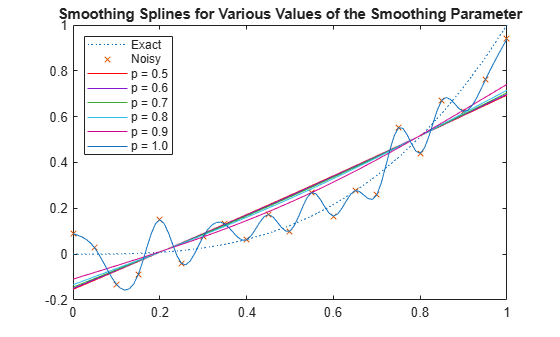We see that the smoothing spline can be very sensitive to the choice of the smoothing parameter. Even for `p` = 0.9, the smoothing spline is still far from the underlying trend, while for `p` = 1, we get the interpolant to the (noisy) data.

In fact, the formulation used by `csapi` (p.235ff of A Practical Guide to Splines) is very sensitive to scaling of the independent variable. A simple analysis of the equations used shows that the sensitive range for `p` is around `1/(1+epsilon)`, with `epsilon := h^3/16`, and `h` the average difference between neighboring sites. Specifically, you would expect a close following of the data when `p = 1/(1+epsilon/100)` and some satisfactory smoothing when `p = 1/(1+epsilon*100)`.

The plot below shows the smoothing spline for values of `p` near this magic number `1/(1+epsilon)`. For this case, it is more informative to look at `1-p` since the magic number, `1/(1+epsilon)`, is very close to 1.

```epsilon = ((xi(end)-xi(1))/(numel(xi)-1))^3/16; 1 - 1/(1+epsilon)```
```ans = 7.8124e-06 ```
```plot(xi,yi,':',xi,ybad,'x') hold on labels = cell(1,5); for j=1:5 p = 1/(1+epsilon*10^(j-3)); yy(j,:) = csaps(xi,ybad,p,xxi); labels{j} = ['1-p= ',num2str(1-p)]; end plot(xxi,yy) title('Smoothing Splines for Smoothing Parameter Near Its ''Magic'' Value') legend( [{'Exact', 'Noisy'}, labels], 'Location', 'NorthWest' ) hold off```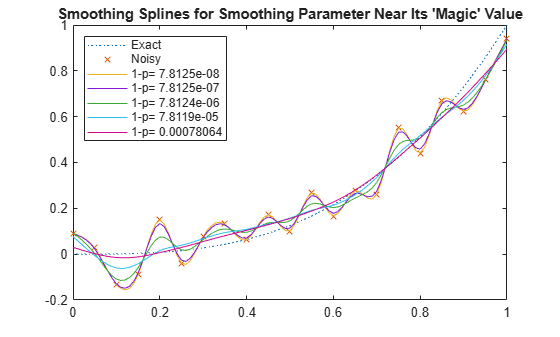In this example, the smoothing spline is very sensitive to variation of the smoothing parameter near the magic number. The one farthest from 1 seems the best choice from these, but you may prefer the one beyond that.

```p = 1/(1+epsilon*10^3); yy = csaps(xi,ybad,p,xxi); hold on plot( xxi, yy, 'y', 'LineWidth', 2 ) title( sprintf( 'The Smoothing Spline For 1-p = %s is Added, in Yellow', num2str(1-p) ) ) hold off```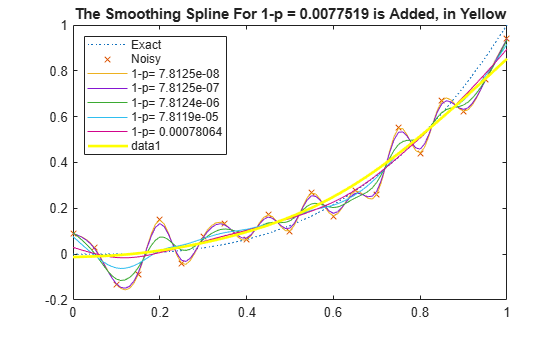You can also supply `csaps` with error weights, to pay more attention to some data points than others. Also, if you do not supply the evaluation sites `xx`, then `csaps` returns the ppform of the smoothing spline.

Finally, `csaps` can also handle vector-valued data and even multivariate, gridded data.

### The SPAPS Command

The cubic smoothing spline provided by the command `spaps` differs from the one constructed in `csaps` only in the way it is selected. Here is an abbreviated version of the documentation for `spaps`:

` SPAPS Smoothing spline.`

` [SP,VALUES] = SPAPS(X,Y,TOL) returns the B-form and, if asked,`

` the values at X, of a cubic smoothing spline f for the given`

` data (X(i),Y(:,i)), i=1,2, ..., n.`

` The smoothing spline f minimizes the roughness measure`

` F(D^2 f) := integral ( D^2 f(t) )^2 dt on X(1) < t < X(n)`

` over all functions f for which the error measure`

` E(f) := sum_j { W(j)*( Y(:,j) - f(X(j)) )^2 : j=1,...,n }`

` is no bigger than the given TOL. Here, D^M f denotes the M-th`

` derivative of f. The weights W are chosen so that E(f) is the`

` Composite Trapezoid Rule approximation for F(y-f).`

` f is constructed as the unique minimizer of`

` rho*E(f) + F(D^2 f),`

` with the smoothing parameter RHO so chosen so that E(f) equals`

` TOL. Hence, FN2FM(SP,'pp') should be (up to roundoff) the same`

` as the output from CPAPS(X,Y,RHO/(1+RHO)).`

### Tolerance vs. Smoothing Parameter

It may be easier to supply a suitable tolerance for `spaps` than the smoothing parameter `p` required by `csaps`. In our earlier example, we added uniformly-distributed random noise from the interval `0.3*[-0.5 .. 0.5]`. Hence, we can estimate a reasonable value for `tol` as the value of the error measure `e` at such noise.

`tol = sum((.3*(rand(randomStream, size(yi))-.5)).^2);`

This plot shows the resulting smoothing spline constructed by `spaps`. Note that the error weights are specified to be uniform, which is their default value in `csaps`.

```[sp,ys,rho] = spaps(xi,ybad,tol,ones(size(xi))); plot(xi,yi,':',xi,ybad,'x',xi,ys,'r-') title( sprintf( 'Clean Data, Noisy Data, Smoothed Values (1-p = %s )', num2str(1/(1+rho)) ) ); legend( {'Exact','Noisy','Smoothed'}, 'location', 'NorthWest' )```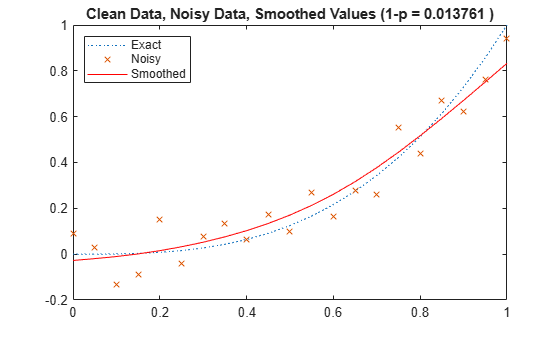The figure title shows the value of `p` you would use in `csaps` to obtain exactly this smoothing spline for these data.

Here, in addition, is the smoothing spline provided by `csaps` when not given a smoothing parameter. In this case `csaps` chooses the parameter by a certain ad hoc procedure that attempts to locate the region where the smoothing spline is most sensitive to the smoothing parameter (similar to the earlier discussion).

```hold on plot(xxi,fnval(csaps(xi,ybad),xxi),'-') title('Clean Data, Noisy Data, Smoothed Values') legend({'Exact' 'Noisy' 'spaps, specified tolerance' ... 'csaps, default smoothing parameter'}, 'Location', 'NorthWest' ) hold off```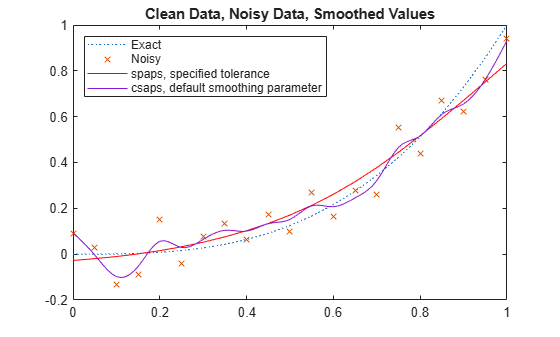### CSAPS vs. SPAPS

The `csaps` and `spaps` commands differ in the way in which you specify a particular smoothing spline, via a smoothing parameter vs. a tolerance. Another difference is that `spaps` can provide a linear or a quintic smoothing spline, in addition to the cubic smoothing spline.

The quintic smoothing spline is better than the cubic smoothing spline in the situation when you would like the second derivative to move as little as possible.